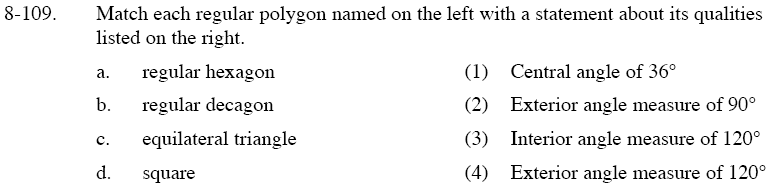Home > GC > Chapter 8 > Lesson 8.3.3 > Problem8-109

8-109.

Match each regular polygon named on the left with a statement about its qualities listed on the right. Homework Help ✎

 a. regular hexagon 1. Central angle of 36° b. regular decagon 2. Exterior angle measure of 90° c. equilateral triangle 3. Interior angle measure of 120° d. square 4. Exterior angle measure of 120°$\text{Interior angle}=\frac{180\degree (n-2)}{n}=\frac{180\degree (6-2)}{6}$

$\text{Central angle}=\frac{360\degree}{10}$

$\text{Exterior angle}=\frac{360\degree}{3}$

$\text{Exterior angle}=\frac{360\degree}{4}$